# SOF - NSO Level 2 Sample Papers for Class 10

Resources:

Class 10 sample paper & practice questions for the level 2 of National Science Olympiad (NSO) are given below. Syllabus for level 2 is also mentioned for these exams. You can refer these sample paper & quiz for preparing for the exam.Q.1 Q.2 Q.3 Q.4 Q.5 Q.6 Q.7 Q.8 Q.9 Q.10
 Q.1 An excess of dilute sulphuric acid reacts with both aqueous barium hydroxide and aqueous barium chloride respectively. In what way are the two reactions same? a) A gas is evolved b) An insoluble salt is formed c) Their final pH is 7 d) A base is produced
 Q.2 Four resistors of 2 Ω each are joined end to end to form a square ABCD. Calculate the equivalent resistance of the combination between any two adjacent corners.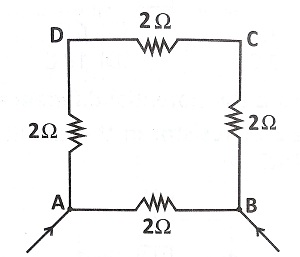a) 1.5 Ω b) 3.0 Ω c) 4.5 Ω d) 6.0 Ω
 Q.3 The power of a lens is + 2.5 D. What kind of lens is it and what is its focal length? a) Concave lens, 100 cm b) Concave lens, 40 cm c) Convex lens, 40 cm d) Convex lens, 50 cm
 Q.4 Which of the given reactions is an odd one? a) Carbon + Oxygen → Carbon dioxide b) Hydrogen + Chlorine → Hydrogen chloride c) Glucose → Carbon dioxide + Water d) Sulphur + Oxygen → Sulphur dioxide
 Q.5 What is the charge flowing through a resistor R in 4 s?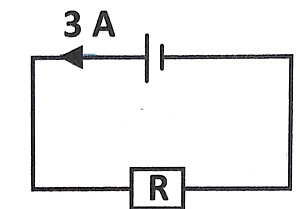a) 3 C b) 6 C c) 12 C d) 18 C
 Q.6 Observe the given circuit. Find the current passing through 6 Ω resistor.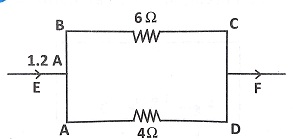a) 0.72 A b) 0.80 A c) 0.48 A d) 0.62 A
 Q.7 Which of the following increases the turning speed of a simple DC motor? (i) An increase in the number of turns of the coil. (ii) An increase in the input of the DC voltage. (iii) The inserting of a copper core in the coil. a) Only (i) and (ii) b) Only (i) and (iii) c) Only (ii) and (iii) d) (i), (ii) and (iii)
 Q.8 Which of the field patterns given below is valid for both electric and magnetic fields? a)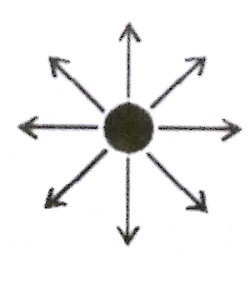b)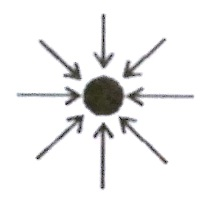c)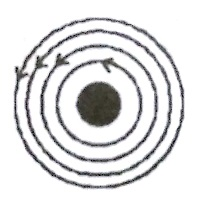d)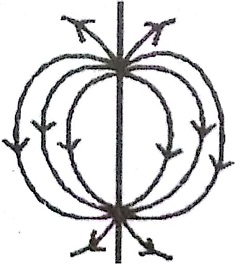Q.9 In an experiment, four resistance wires made from the same material are connected in between the terminals P and Q in the circuit as shown below. The length and diameter of the wires are different. Which wire will give the smallest reading on the ammetre?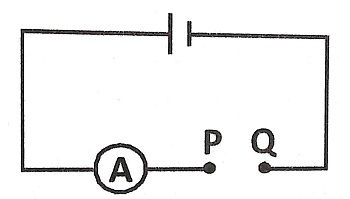a) Length - 0.5 m, Diameter -0.5 mm b) Length - 0.5 m, Diameter - 0.05 mm c) Length - 5 m, Diameter - 0.5 mm d) Length - 5 m, Diameter - 0.05 mm
 Q.10 A current carrying wire in a magnetic field results in ________. An electric force Magnetic induction A motor effect a) Only (i) b) Only (ii) c) Only (iii) d) None of the above